# 基于微带馈电的平面单极子超宽带天线

2014-10-23 来源：互联网 字号：

1、引言

2、天线结构

(1)介质基片：W=25，L=30，H1=1.9;(2)地板：L1=14，H2=0.08，g=1;(3)锥形渐变线：W1=3，W2=8，S=3.6，S1=1.6，L3=2.5，L4=3.5，L5=9，H=0.08;(4)辐射臂：W3=25，W4=15，r1=5，r2=5。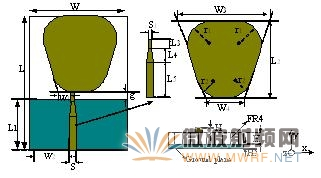3、天线的仿真结果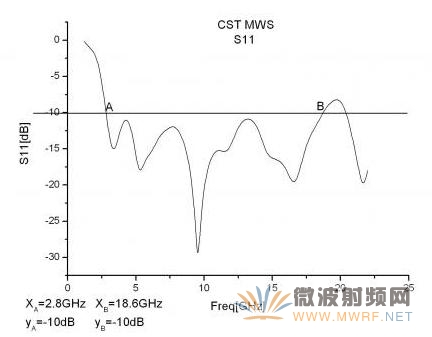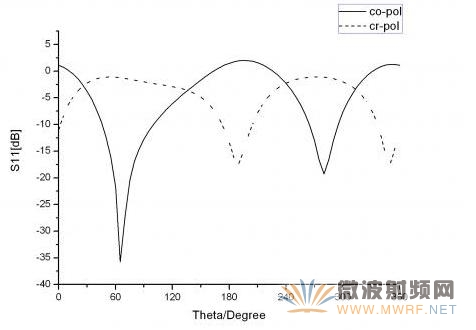6GHz E 平面 (Y-Z 平面)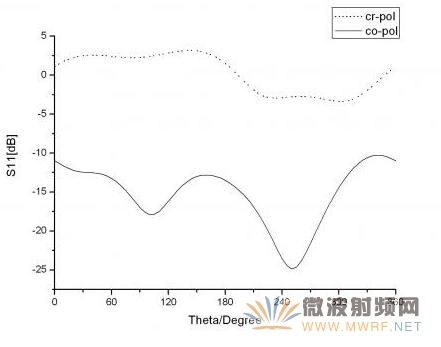6GHz H 平面(Y-X 平面)图3(A)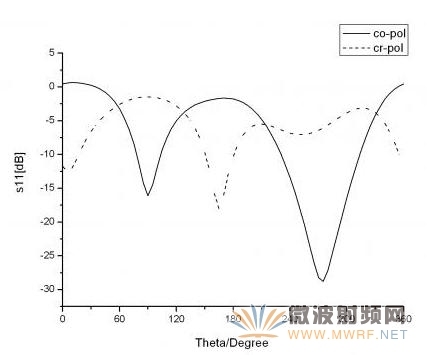9 GHz E 平面(Y-Z 平面)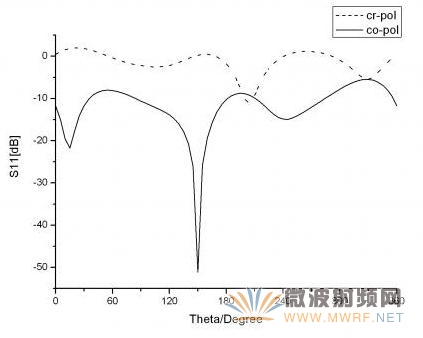9 GHz H 平面 (Y-X 平面)图3 (B)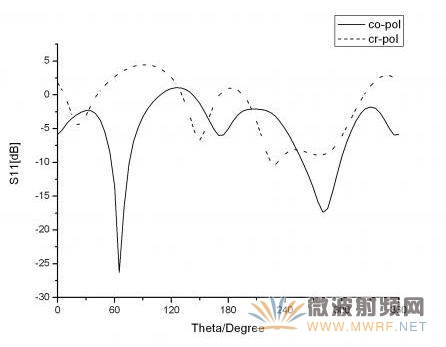16 GHz E 平面 (Y-Z 平面)16 GHz H 平面 (Y-X 平面)图3 (C)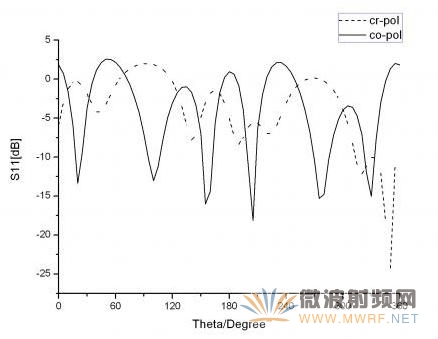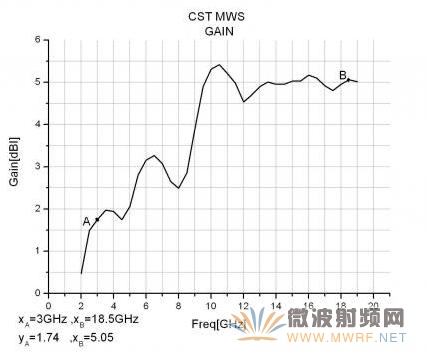4、不同参数与几何结构对天线的影响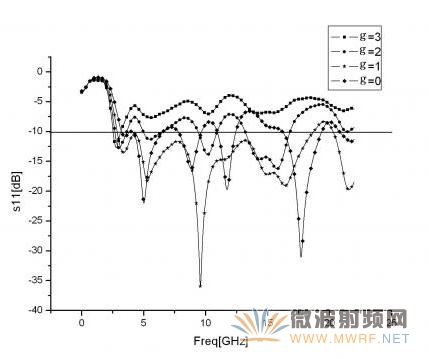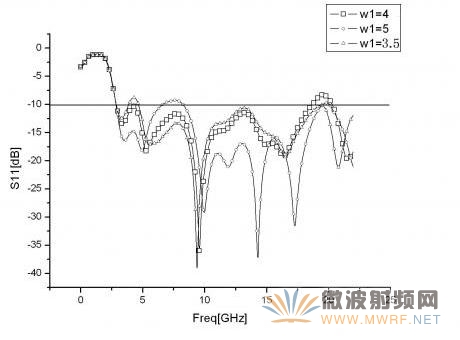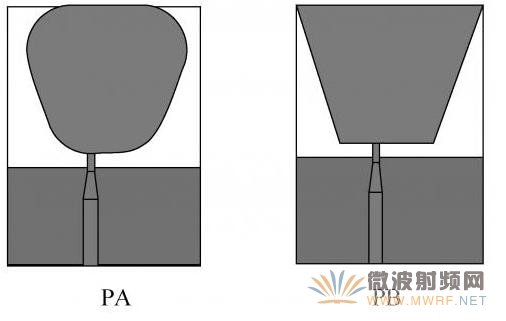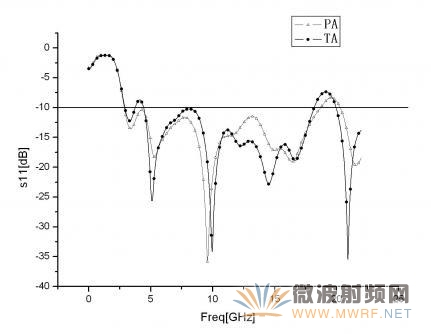5、结论